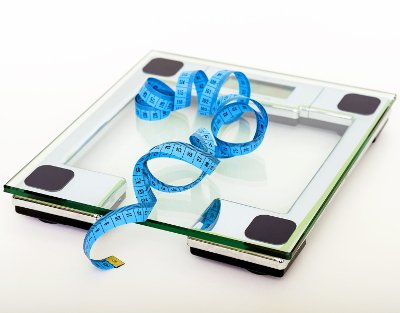# Body Adiposity Index (BAI) Calculator

Share:

Enter your height and hip circumference. Then click the "Calculate BAI" button.

*
*
*
*
ft
Feet
in
Inches
cm
Centimeters
*
In
Inches
Cm
Centimeters

Similar Fitness Calculators

## Body Adiposity Index (BAI) CalculatorThis on-line ABSI calculator takes your height and hip circumference and calculates the BAI value. Then, based on the selected ethnicity, age and gender, it will identify the Health risk classification in which the body fat percentage comes under.

### Using the BAI calculator

1. Select the unite of measurement. Either Metric (Kilogram, centimeter, etc..) or U.S.A Standard / Imperial (Pound, Inches, Feet, etc..).
3. Select your Age. You can select from 10 years to 79 years.
4. Enter your Height either in centimeters or in feet.
5. Enter your Hip Circumference. Follow the guidelines below to measure the hip circumference.
6. Then, click the Calculate BAI button. The BAI value will be calculated and the result will be displayed in the box below the button.

### Guide for measuring hip circumference

1. Wear less cloth as possible
2. Stand in front of a full body mirror.
3. Keep the feet close together with little space between them.
4. Place the tape around the maximum circumference of the buttocks. Make sure the tape is in horizontal position.
5. Breath normally. Take the measurement after the exhaust.

Body Adiposity Index (BAI) is one among the attempts to identify the obesity of the human body by calculating the percentage of body fat using height and hip circumference. BAI was formulated in order to overcome the shortfalls of the widely used Body Mass Index (BMI). But according to some researched, BAI is not more accurate than BMI (see reference below).

### BAI Formula

Formula to calculate the BAI:

BAI = (Hip ÷ Height1.5) - 18

• Hip = Hip Circumference in centimeters (cm).
• Height = Height in meters (m).
• BMI = Body Mass Index.

### Risk Ranges Of Body Fat Percentage Based On Gender And Ethnicity

(This body adiposity index risk classification is based on the journal "Healthy percentage body fat ranges" by Dympna Gallagher, Steven B Heymsfield,.... See reference below)

#### Women

Age Body Fat % Range (Based On Ethnicity) Risk Level
Asian African American White
20 to 39 Yrs Less than 25% less than 20% Less than 21% Underweight
Between 25% and 35% Between 20% and 32% Between 21% and 33% Healthy
Between 35% and 40% Between 32% and 38% Between 33% and 39% Overweight
Above 40% Above 38% Above 39% Obese
40 to 59 Yrs Less than 25% less than 21% Less than 23% Underweight
Between 25% and 36% Between 21% and 34% Between 23% and 35% Healthy
Between 36% and 41% Between 34% and 39% Between 35% and 41% Overweight
Above 41% Above 39% Above 41% Obese
60 to 79 Yrs Less than 26% less than 23% Less than 25% Underweight
Between 26% and 36% Between 23% and 35% Between 25% and 38% Healthy
Between 36% and 41% Between 35% and 41% Between 38% and 43% Overweight
Above 41% Above 41% Above 43% Obese

#### Men

Age Body Fat % Range (Based On Ethnicity) Risk Level
Asian African American White
20 to 39 Yrs Less than 13% less than 8% Less than 8% Underweight
Between 13% and 23% Between 8% and 20% Between 8% and 21% Healthy
Between 23% and 28% Between 20% and 26% Between 21% and 26% Overweight
Above 28% Above 26% Above 26% Obese
40 to 59 Yrs Less than 13% less than 9% Less than 11% Underweight
Between 13% and 24% Between 9% and 22% Between 11% and 23% Healthy
Between 24% and 29% Between 22% and 27% Between 23% and 29% Overweight
Above 29% Above 27% Above 29% Obese
60 to 79 Yrs Less than 14% less than 11% Less than 13% Underweight
Between 14% and 24% Between 11% and 23% Between 13% and 25% Healthy
Between 24% and 29% Between 23% and 29% Between 25% and 31% Overweight
Above 29% Above 29% Above 31% Obese